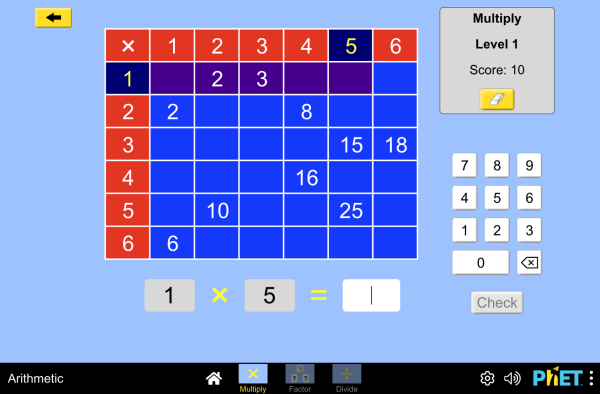## Arithmetic by PHeTClick to Run

### Topics

• Multiplication
• Division
• Factoring

### Description

Remember your multiplication tables? Practice your multiplication, division, and factoring skills with this exciting game. No calculators allowed!

### Sample Learning Goals

• Explain how multiplication tables help understand multiplication, factoring, and division.
• Use an array model to understand multiplication, factoring, and division.
• Increase accuracy in multiplying, factoring and dividing.
• Develop multiple strategies for arithmetic problems.

Phet